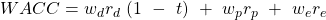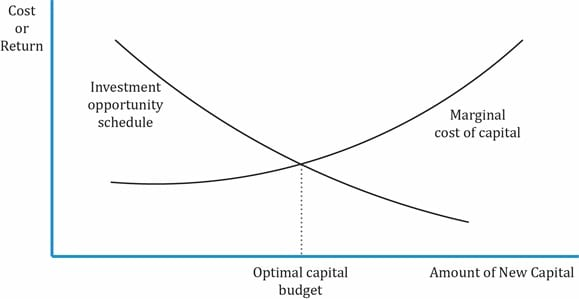IFT Notes for Level I CFA® Program
IFT Notes for Level I CFA® Program

# Part 1

## 1.  Introduction

This reading defines what is cost of capital, methods to estimate the cost of capital, and why estimating the cost of capital accurately is important, both for decision-making by a company’s management and for valuation by investors. Estimating the cost of capital is a complex process which requires many assumptions.

## 2.  Cost of Capital

Cost of capital is the rate of return that the suppliers of capital require as compensation for their contribution of capital. Assume a company decides to build a steel plant and needs money or capital for it. Investors such as bondholders or equity holders will lend this capital to the company. Suppliers of capital will be motivated to part with their money for a certain period of time if the money invested can earn a greater return than it would earn elsewhere. In short, investors will invest if the return (IRR) is greater than the cost of capital.

Riskier projects will have a higher cost of capital.  A company has access to several sources of capital such as issuing equity, debt, or instruments that share characteristics of both debt and equity. Each source becomes a component of the company’s funding and has a specific cost associated with it called the component cost of capital.

The cost of capital is the rate of return expected by investors for average-risk investment in a company. One way of calculating this cost is to determine the weighted average cost of capital (WACC), which is also called the marginal cost of capital. It is called marginal because it is the additional or incremental cost a company incurs to issue additional debt or equity.

Three common sources of capital are common shares, preferred shares, and debt. WACC is the cost of each component of capital in the proportion they are used in the company.where
wd = proportion of debt that the company uses when it raises new funds
rd = before-tax marginal cost of debt
t = company’s marginal tax rate
wp = proportion of preferred stock the company uses when it raises new funds
rp = marginal cost of preferred stock
we = proportion of equity that the company uses when it raises new funds
re = the marginal cost of equity

Example

IFT has the following capital structure: 30 percent debt, 10 percent preferred stock, and 60 percent equity. The before-tax cost of debt is 8 percent, cost of preferred stock is 10 percent, and cost of equity is 15 percent. If the marginal tax rate is 40 percent, what is the WACC?

Solution:

WACC = (0.3) (0.08) (1 – 0.4) + (0.1) (0.1) + (0.6) (0.15) = 11.44 percent

Note: Before-tax cost of debt is given. Do not forget to calculate the after-tax cost.

Example

Machiavelli Co. has an after-tax cost of debt capital of 4 percent, a cost of preferred stock of 8 percent, a cost of equity capital of 10 percent, and a weighted average cost of capital of 7 percent. Machiavelli Co. intends to maintain its current capital structure as it raises additional capital. In making its capital budgeting decisions for the average risk project, what is the relevant cost of capital?

Solution:

The relevant cost of capital is 7%. The WACC using weights derived from the current capital structure is the best estimate of the cost of capital for the average risk project of a company.

### 2.1.     Taxes and the Cost of Capital

Notice that in the equation for WACC, we consider taxes only for debt. This is because payments to equity shareholders in the form of dividends are not tax-deductible. On the other hand, interest costs are tax-deductible in some jurisdictions; they pass through the income statement and provide a tax shield. If interest expense is not tax deductible then the tax rate applied is zero and effective marginal cost of debt is equal to cost of debt (rd). Let us see the effect on net income in the example below.

A company pays 10% interest on capital raised. On the left hand side of the table below, you see that the interest is tax deductible. On the right hand side, the interest is not tax deductible. So the tax expense on the LHS is 16, which is 4 less than that on the RHS. The savings on taxes consequently reflect in the net income as well. The actual cost of debt is 6% when it is tax-deductible instead of 10%.

 Calculation of net income assuming interest is tax-deductible Calculation of net income assuming interest is not tax-deductible Revenue                         100 Revenue                           100 Operating Expenses    50 Operating Expenses       50 Interest                           10 EBT                                    50 EBT                                 40 Tax expense (40%)          20 Tax Expense (40%)      16 Interest Expense              10 Net Income                    24 Net Income                        20

After-tax cost of debt = Before-tax cost of debt x (1 – tax rate)

### 2.2.     Weights of the Weighted Average

Any company raising capital always has a target capital structure and raises capital in line with this structure. This information is typically internal to a company, and not available to an analyst. In such cases where the information is not available, an analyst should use the market values of debt and equity as a proxy for the target capital structure (instead of using book values).

Let us take a simple scenario where the capital structure consists of only debt and equity. So, the WACC is . In the table below, you can see the book value and market value of debt is the same. But for equity they are different. While computing WACC, you should use the market values for the weights and ignore the book values. So the weight of debt is 0.2 and the weight of equity is 0.8.

Book Value                 Market Value

Debt                            20                                             20

Equity                         40                                             80

Weights should be based on:

• Market values.
• Target capital structure: when data is given for the current capital structure and target capital structure, use the target capital structure as this is the proportion the company is striving to achieve.

In the absence of explicit information about a firm’s target capital structure, one may estimate it using one of the following approaches:

• Current capital structure based on market value weights for the components (most common method).
• Trend in the firm’s capital structure or statements made by management regarding capital structure policy.
• Average of comparable companies’ capital structures as the target capital structure.

Example

You gather the following information about the capital structure and before-tax component costs for a company. The company’s marginal tax rate is 40 percent. What is the cost of capital?

 Capital component Book value (in 000) Market Value (in 000) Component cost Debt $100$90 8% Preferred stock $20$20 10% Common stock $100$300 14%

Solution:

Use the market value to calculate the weights of each component.

wd = 90/410 = 0.22

wp = 20/410 = 0.05

we = 0.73

WACC = 0.22 (8) (0.6) + 0.05 (10) + 0.73 (14) = 11.78%

### 2.3.     Applying the Cost of Capital to Capital Budgeting and Security Valuation

A company’s marginal cost of capital may increase when additional capital is raised. Similarly, the return on investment decreases as a company invests in additional opportunities. Assume a company has borrowed money from a bank for a project. When it borrows additional money, the cost of capital increases as the riskiness of the company or the investment goes up.

This relationship between investment opportunity schedule (IOS) and marginal cost of capital (MCC) is depicted in the graph below. The following points can be inferred:

• The return on investment is greater than the cost of capital to the left of the optimal point; in this area, it is prudent for the company to borrow more money to invest in projects.
• The return is less than the cost of capital to the right of the optimal capital budget, i.e., in this area it is not profitable for the company to raise more money.
• The optimal capital budget is the amount of capital at which the marginal cost of capital is equal to the marginal return from investing, or the point at which IOS intersects MCC schedule.How WACC or MCC is used in capital budgeting:

WACC or MCC is used as the discount rate to compute NPV for average risk projects.

NPV = present value of inflows – present value of outflows

This assumes the target capital structure stays constant and the project has the same risk as that of the company. Adjustments to the cost of capital are necessary when a project differs in risk from the average risk of a firm’s existing projects. The discount rate should be adjusted upwards for higher risk projects and downwards for lower risk projects.